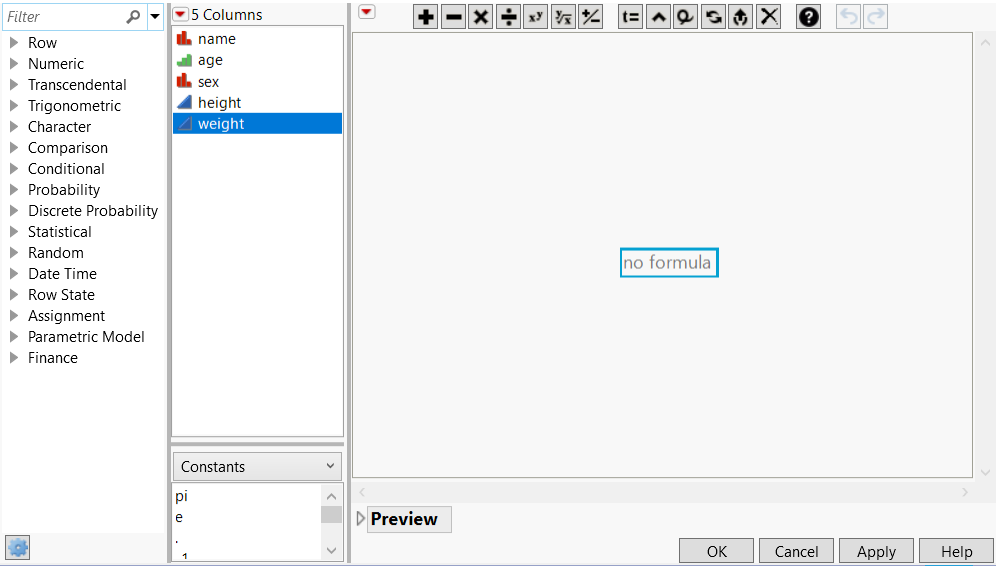Using JMP > Create Formulas in JMP > Insert Constants in Formulas
Publication date: 03/23/2021

# Insert Constants in Formulas

Formulas can be simple assignments of numeric, character, or row state constants, or they can contain complex evaluations based on conditional clauses. Constants include commonly used numeric terms, such as e, pi, -1, 0, 1, and 2. There are two ways to add a constant value to a formula:

Enter them manually using the keyboard

Select them from the list in the middle pane, as shown in Figure 7.9.

Figure 7.9 The Formula Element Browser with Constants SelectedTo add constants to a formula, follow these steps:

1. Open the Formula Editor by right-clicking a column name in the data table and selecting Formula.

2. Select a box in the formula editing area by clicking it. (It is selected when there is a blue outline around it.)

3. Either type in a number or select Constants in the middle pane, as shown in Figure 7.9. Then click a value in the list that appears: 0, 1, 2, -1, pi, e. The value appears in the outlined box.

4. Complete the remainder of the formula using the keypad and functions. (See Refer to Data Table Values in Formulas, Add Operators to JMP Formulas, and Use Functions in Formulas.)

Note: Double-clicking or pressing Alt and clicking the constant replaces the portion of the formula that is selected.

Want more information? Have questions? Get answers in the JMP User Community (community.jmp.com).